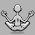## Monday, 30 January 2012

### Filtering Arrays

Stephen Blair has been posting some great articles on the eX-SI Support Blog about how to create array patterns i.e ordered sequences like (0,1,2,3,0,1,2,3) or (0,0,0,1,1,1,2,2,2). The creation of this type of sequence can be useful in creating  the points of a grid and also for avoiding the use of repeat loops in certain circumstances.

On the XSI mailing list a few weeks back Dan Yargici posted a question about how to reconfigure a simple non-regular pattern like (1,1,5,5,5,5,8,9,9,9,10,10) into (1,1,2,2,2,2,3,4,4,4,5,5). In the resultant thread Martin Chatterjee came back with a brilliant solution without using repeats. Martin's solution touched on some of the inherent functionality in ICE arrays that's worth expanding upon.

I'm going to try and illustrate some of these with a sample scene that contains an ICE tree that shows several different methods for firstly creating a pattern array and then using that pattern to manipulate an array. If that all sounds abstract it's based on Dan's problem above but with the added wrinkle that the initial pattern is not numerically ascending i.e. it looks something like this: (8,8,2,2,2,14,3,3,1,16,11,11).

The first task is to identify the points at which the pattern changes e.g. when the preceding item in the array is not the same as the current one. There are a couple of ways of doing this illustrated in the scene, but it's the first method that illustrates our first point.You can create an identical array and use the insert in array node to add zero at index zero. Although the arrays are now of different lengths you can subtract the two arrays. Wherever the resultant value is non-zero you can mark the array with a boolean. So, here's the first key point about ICE arrays:
• ICE arrays do not have to be the same size for operations (e.g. subtraction)
The subtraction calculation will proceed until one of the arrays runs out and the resultant array will be truncated to that length. It seems like an esoteric point in the documentation, but it's genuinely useful.

You should now have an array consisting of (1,0,1,0,0,1,1,0,1,1,1,0) i.e a series of boolean values. Each 1 represents the starting point of a new digit in the pattern. In this form it's not much use but if you convert the booleans to integers and then multiply by the subindex array you get a list of indices in the original array where the pattern changes - the actual constituent numbers of the pattern. Multiplying subindices by a boolean (converted to integer) is a neat way of isolating the array indices you're interested in.In this case we get (0,0,2,0,0,5,6,0,8,9,10,0).

It's still not entirely usable, though, since it also contains a lot of zero entries. If we were to run this new list through a select in array node we would be picking the zero index entry multiple times. Which brings us to our second point:
•  If you feed an index array into the index port of select in array or remove from array you get an equally sized** array as your output*. This output array can contain multiple duplicates of an index if you require it. (* The values output of remove from array). (**Provided all the input indices are valid - more on this below.)
What this means is that if you feed (0,0,0,0,0) into the index port of select in array you will get back an equally sized array with each index of the new array containing the value of index zero from the old array.

We need to either remove the zero entries or, at least, filter them out in such a way that ICE does not action them. Here's where, I guess, the main trick of this article lies:
• ICE has been optimised to ignore invalid index entries for arrays
By 'invalid index', I mean any entry for an array index that is either negative or exceeds the array size minus one. There are three key nodes where this is relevant: remove from array, select in array and set in array. If for example, you feed a remove from array node the indices (-1,-5, 99, 0,1,2,3) and your array size is 10 then only the valid indices will be removed (0,1,2,3). In conventional scripting/programming you would never normally use an invalid index value without risking a serious crash. In ICE there's no need for any repeat loops or detours in the data flow to remove them.

So, in our case, with an array like (0,0,2,0,0,5,6,0,8,9,10,0) where all we're interested in are the non-zero entries we just need to make the zeros into invalid indices (negatives or values that exceed the array size minus one i.e. (-1,-1,2,-1,-1,5,6,-1,8,9,10,-1). This new array can be fed into a select in array node and will only select the following indices (2,5,6,8,9,10). Effectively, you've filtered the array without a repeat loop.The sample scene shows a couple of different ways of doing this.

The final point to make is that two of the array nodes - insert in array and set in array - can each take two array inputs. One for the indices to be affected and one for the values to be utilised at those indices. As above, those arrays can be different lengths - the processing will truncate the length of the longest array to the shortest. What's more, any invalid indices will be ignored and thus any parallel value (ie. one at the same index) in the value array will  also be ignored.

It's dry and it's boring but being aware of these kinds of optimisations and behaviours of the ICE array nodes can be very useful as, I think, Martin's solution demonstrated.

Sample scene is here.

#### 1 comment:

1.Very clever lateral thinking. Lots of good tips, thanks Jules.

(I have to admit, in these circumstances, I usually give up and write it in C++)